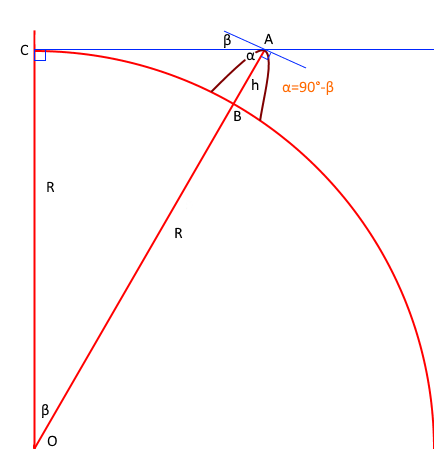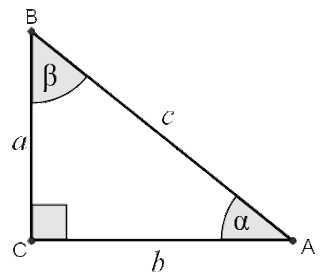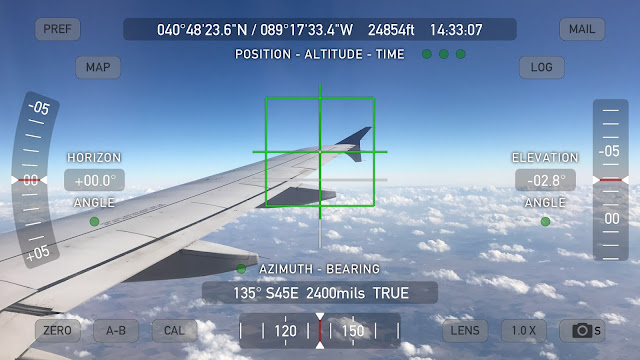This blog is for nonprofit, educational purposes - media is incorporated for educational purposes as outlined in § 107 of the U.S. Copyright Act.

## Can we know the shape of the Earth?

or

### How Al-Biruni Calculated the Circumference of the Earth Using a Mountain in the 11th century

As you might recall from my earlier posts, I've used the Sun at local Solar Noon (when the Sun is at its zenith) on the Equinox as a way to know the shape of the Earth.  But all we need is some point that is high enough to be seen at some significant distance and Mountain peaks are much easier to experiment with than a moving Sun.

If the ground is a flat plane then we can simply triangulate using the mountain peak at different distances and get the SAME answer for the height using simple trigonometry:Figure 1

For example, if the ground was flat, we could find h by simply measuring our angle α at two different distances, where Δd is the distance between the two observation points using the formula:

Δd * tan(α₀) * tan(α₁)
h = ----------------------
tan(α₁) - tan(α₀)

### Can you prove that?

This seems complex but lets think about what the tangent function REALLY does. You put in an ANGLE and you get back the matching SLOPE.  And SLOPE is simply RISE over RUN (rise/run). That's why we have the relationship: tan(θ) = opposite/adjacent -- once you understand that it is simply converting an angle into a slope I think things get so much simpler.  All we are really doing here is a unit conversion.

And since it is rise/run we can expand this equation by replacing the tangent function with the equation for each of our slopes and we get:

(d₀-d₁) * (h/d₀) * (h/d₁)
-------------------------
h/d₁ - h/d₀

Now what we want to do is prove (mathematically) that this equation would give us the value for h if we plugged in all the values.  To do that we need to show that we can simplify the equation and everything will cancel out, leaving us the value for h at the end.

First, let's multiply out our numerator and move the denominator over to keep things easier to read (dividing by something is the same as multiplying by the inverse):

h*h*(d₀-d₁)          1
------------- * ------------
d₀ * d₁       h/d₁ - h/d₀

Next, we can join the denominator on the left by multiplying both fractions by the Least Common Denominator (which is [d₀*d₁], and simplify our new denominator):

h*h*(d₀-d₁)      d₀ * d₁
------------- * ----------
d₀ * d₁       h*(d₀-d₁)

Now we can see that [d₀ * d₁] cancels out leaving:

h*h*(d₀-d₁)
-------------
h*(d₀-d₁)

And we can cancel out the common term [h*(d₀-d₁)] from the numerator and denominator which leaves us with just:

h

So we have proven mathematically that our starting (slightly complex) equation will give us the value we need. Our problem is we don't know the value of h to find h/d₀ directly but since we do know what the value of h/d₀ is (because we have the angle and h/d₀=tan(α₀)) we can plug in the values and get the answer back out of the equation.  So we don't even need the distance to the mountain in this case, just the distance between our two observations and the angle to the peak is sufficient to calculate the height AS LONG AS our ground plane is flat.

However, when you try this over longer distances, you find that it doesn't quite work. As you get further away from the mountain the mountain appears to shrink. The further away you get the more it shrinks.  If you try it with a streetlamp you'll find that it works very well because the ground is flatter than your measurement error over short distances.  But with mountains or tall buildings you begin to measure the curvature of the Earth appearing to make the object shorter and shorter with distance.

And the amount it shrinks is pretty consistent no matter which direction you go from the mountain so we know the curvature is pretty even in all directions and at all points around the Earth where we try to measure tall objects.

It is because of this, almost** equal shrinkage in all directions, at all points around the Earth, that we can conclude the Earth is a spheroid (and with better measurement accuracy we can tell it isn't EXACTLY equal in all directions but we can tell that it's a little bit oblate or squished making it a little bit wider around the equator than around the poles).

** On a sphere it would be equal, on our slightly oblate/squished ellipsoid Earth it varies slightly, mainly with latitude.

So what we find is that our ground is NOT flat because our angles keep changing with distance as the ground is rotating underneath us, throwing off our calculation:Figure 2

This doesn't mean that triangulation is useless (but it fairly conclusively, confirms the shape of the Earth as being curved).  But what we need to be able to do is take our measurements from close enough to the mountain and from some height so we can still see the same base point on the mountain so that our baseline is flat, based on a direct line-of-sight.

Ok, so we can use this to calculate the height of the mountain as long as we make sure each observation point measures the angle between the same spot at the base of the mountain and the peak AND we now know that the Earth is curved.

What Al-Biruni figured out in the 11th century was that if he could find the height of the mountain peak as above (using angles and distances) AND find the angle between a level on the peak and the horizon (shown as angle β below) then this would also be the angle made at the center of the circle, and from this he could estimate the Radius of the Earth, and with the Radius of the Earth it is easy to find the circumference (r·2π).

Here is the geometry that Al-Biruni had in mind, at point A at the peak of the mountain we measure the angle of the dip to the horizon (greatly exaggerated here, it will usually be a smaller angle):Figure 3

Instead of a tangent, this time we're going to look at the sin function.

What we know about sin is that it is also a ratio, just like our tangent function, consider a right triangle:Figure 4

sin(x) = opposite / hypotenuse

What 'opposite' means here is the length of the side opposite the angle in question - so we have the following relationships:

sin(A) = a/c
sin(B) = b/c
sin(C) = c/c

From this it is easy to show that the Law of Sines holds:

a        b       c
------ = ----- = ------
sin(A)   sin(B)  sin(C)

By simply expanding our formula, for example:

b    b c
--- = -*- = c
b/c   1 b

We also know that the sum of all the angles must equal 180° and that one of our angles is 90° so when we measure the angle β we also can know that α is simply 90°-β and we also know that sin(90°-β) = cos(β) [I'll leave this as an exercise for the reader].

Now armed with these identities we can write out the equations for this diagram of the Earth using our Law of Sines:

b       c
----- = ------
sin(B)  sin(C)

And we substitute in our known values, where:

b = R
sin(B) = sin(90°-β) = cos(β)
c = R+h
sin(C) = sin(90°) = 1

So that gives us a starting equation where we can solve for R.

R
------- = R + h
cos(β)

Isolate R to one side by subtracting R(cos(β)/cos(β)) from both sides, you will see why we added the (cos(β)/cos(β)) in the next step:

R      R cos(β)           cos(β)
------- - -------- = R - h - --------
cos(β)    cos(β)             cos(β)

Which simplifies to:

1
R(------ - 1) = h
cos(β)

We can then multiply both sides by the inverse, or 1/(1/cos(β)-1) rewritten as cos(β)/(1-cos(β)) which gives us:

cos(β)
R = --------
1-cos(β)

Which can also be written:

h
R = -------- = h/(sec(β)-1)
sec(β)-1

So now we have the portion of our triangle represented by R in terms of the height of our mountain and the angle between level at the top of the mountain and a distant point on the horizon.

Let's say we're up on a Mountain at 2000 meters.  The dip angle to the horizon should be about 1.43546°.   Plugging that into our formula:

2000/(sec(1.43546°)-1) = 6.371×10⁶

### Measurements On A Plane

I took some images during a recent flight using this Theodolite app and got a few good shots.  This was my first attempt at this so I have a whole bunch of shots where I carefully captured 0 tilt along both axes -- and it turns out those are almost useless (lesson learned).Figure 5 - nice, but doesn't measure the horizon dip angle

Which is a nice level photo but what I figured out I wanted to do was let the Theodolite measure the angle to the horizon.  Fortunately, I did just that for a few of the later shots.

There are several challenges in this, first is that the horizon is difficult to observe at higher altitudes due to the atmosphere and secondly, the tilt angle accuracy of an iPhone is limited.  My app only reads out in tenths of a degree so at 10000' we could be ±500 miles Earth Radius.  We really need about 4 decimal places (3.4883°) at 38800 feet but we're only going to get 1 decimal place and maybe we can justify bumping that up a half because we're still slightly above the horizon.

First let's test out our app and make sure it works on the ground.  What I did here is tilt the phone both down and counterclockwise to show that the reticle (showing red here) finds "LEVEL" pretty nicely (I took this at the start of my return flight as I realized I needed a better image to show that the app works pretty well and was properly calibrated - I did not alter the calibration AT ALL, this is the default, 'uncalibrated' accuracy but you don't need to take my word for it, do your own experiments).Figure 6 - reticle marks level even if we tilt the phone
Let's jump into the observations now, as I captured two photos that show what I was hoping to show.  This photo is taken at 38800 feet and I've tilted my phone down 3.4° to place the white center crosshair on the horizon.  I would say that the crosshair is slightly above the horizon so let's say the actual angle is about 3.45° as there are clearly some clouds in the way here.

Plugging these values into our formula we get (I divide by 5280 to convert feet to miles):

38800/(sec(3.45°)-1)/5280 = 4047 miles - so we're 88 miles off the actual value of 3959 miles, well within our measurement error.Figure 7 - with increased contrast to improve horizon view [original]

This next image was taken about 36 minutes later, as we started descending and we're at 24854 feet with a horizon dip angle of about 2.8° and you can see I got below that upper cloud edge in this photo so what we get here is:

24854/(sec(2.8°)-1)/5280 = 3940 miles - so we're just 19 miles off the actual radius of the Earth here.Figure 8 - no contrast adjustment

We've got a decent estimate for the Earth Radius and therefore agreement with the Spheroid/Globe model.  We could further reduce the error introduced by our limit on measuring the angle by taking lots of observations at different altitudes and averaging them.

Next time you are going on a flight  maybe you'll get a better view of the horizon and you can estimate the circumference of the Earth.  Can you beat Al-Biruni's value which was correct to within ~200 miles?

#### 1 comment:

1.That, Sir, is a simple, yet great achievement. Congratulation! :)

Do you have the values of the dip that Albiruni measured? Just curious about Albiruni's accuracy.